Subsections

# Problem Statement and Previous Work

The registration of images of deformable surfaces has received a growing attention over the past decade. Usually, for purely twodimensional registration, as is the case in this paper, smoothness constraints are used to filter out the noise and `fill-in' the optical flow in untextured image areas. These soft constraints are either implicitly incorporated in a parameterized warp or enforced through regularization. In this paper, we focus on direct as opposed to feature-based methods, e.g. (185,145). In the feature-based methods, the warp parameters are estimated from features, such as points, which have first been extracted and matched in the images to register (181). Note that there exist methods that mix both the feature-based and the direct approaches to image registration. See for instance, (102,81).

Direct registration consists in minimizing the pixel value discrepancy. Registration of an image sequence is posed as a set of nonlinear optimization problems, each of which estimatingusing the registration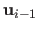of the previous frame as an initial solution. The discrepancy functionis usually chosen as the two-norm of the difference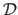between the texture image and the current one, warped towards the texture image, i.e., giving: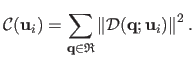(A.1)

Other choices are possible for the cost function, such as the Mutual Information, see e.g. (125,150), or a criterion based on the gradient of images, see (89).

Several algorithms have been proposed to minimize. We classify them in two groups: the Forward Additive algorithms and the Inverse Compositional ones.

## Forward Additive Algorithms

#### Forward Additive Gauss-Newton (FA-GN).

Using an additive update of the parameter vector, i.e.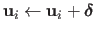, Gauss-Newton can be used in a straightforward manner for minimizing equation (A.1) or in conjunction with complexity tuning schemes as in (16,111) for the TPS warp. The local Gauss-Newton approximation tois given by the first order Taylor expansion in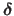of each squared term in equation (A.1):(A.2)

The gradient vector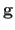is the product of the image gradient vector and of the Jacobian matrix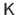of the warp, i.e.. The Gauss-Newton approximation induces a Linear Least Squares minimization problem in. Defining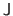as the Jacobian matrix of the error, obtained by stacking the gradient vectors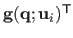for all the pixelsin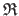, andthe residual error vector, the solution is obtained through the normal equations: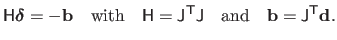(A.3)

The matrixis the Gauss-Newton approximation to the Hessian matrix. Note that,and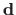depend on. The Jacobian matrixmust be recomputed at each iteration, implying thatmust be recomputed and inverted as well.

#### Forward Additive ESM (FA-ESM).

A second order approximation ofcalled ESM (Efficient Second-order Minimization), theoretically better than the Gauss-Newton one, is proposed in (21). Combined with an additive update of the parameters, it gives:(A.4)

The ESM approximation has been shown to improve the convergence rate, compared to Gauss-Newton, without increasing the computation time per iteration since the gradient vectors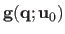are constant.

## Inverse Compositional Algorithms

The major drawback of the two above-presented methods is that the image gradient vector for each pixel inmust be recomputed at each iteration. This is the most expensive step of the process. A major improvement was proposed by (9) with the Inverse Compositional algorithm. The first key idea consists in switching the roles of the texture and of the current images:(A.5)

The second idea is to update the current warp by composition:(A.6)

Using Gauss-Newton for local registration leads to a constant Jacobian matrix and a constant Hessian matrix whose inverse is thus pre-computed. Of course, the inverted warp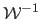exists only if the considered set of warps is a group. For instance, homographic warps are used in the original Inverse Compositional algorithm (9). In this case, inversion is obtained by inverting the associatedmatrix and composition by multiplying those matrices. Several attempts have been made to relax the groupwise requirement for flexible models.

As proposed in (158,75,123), these attempts usually consist in finding the best approximating warp for the pixels of interest in: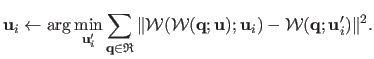(A.7)

In (158,123), the warp is induced by a triangular mesh whose deformations are guided by a parameter vector. This minimization problem is usually solved in two steps. First the vertices in the current image are computed using the assumption of local rigidity. They usually are not in accordance with a model instance in e.g. the case of 3D Morphable Model (158,75). Second, the parameter update is recovered by minimizing a prediction error, i.e. the distance between the updated vertices and those induced by the parameters. This last step may be time consuming since nonlinear optimization is required. Warp inversion is approximated with first order Taylor expansion in (123), while (158) draws on triangular meshes to avoid linearization. By comparison, our method reverts and threads warps in closed-form: it does not require optimization.

Other methods have been proposed to obviate the shortcomings induced by non-groupwise warps. For instance, one may force the solution space to contain diffeomorphic warps (47,103,102). The solution space thus constitutes a group. Requiring the warps to be diffeomorphisms may be an overly strong requirement. This is especially true when the deformations are not too important. Indeed, as noted in (102), with such deformations the estimated warps may be diffeomorphisms even though the solution space contains non-diffeomorphic warps. Besides, such approaches make the use of standard deformation models such as the TPS and the FFD generally impossible. This is one interesting point of the proposed approach in this paper: it proposes an efficient method which is built on top of the most common deformation models for image registration. Finally, enforcing the estimated warps to be diffeomorphisms is often achieved by adding supplementary constraints to an initial forward estimation algorithm. These constraints are generally impractical to design a fast inverse-compositional estimation algorithm.

Contributions to Parametric Image Registration and 3D Surface Reconstruction (Ph.D. dissertation, November 2010) - Florent Brunet
Webpage generated on July 2011
PDF version (11 Mo)